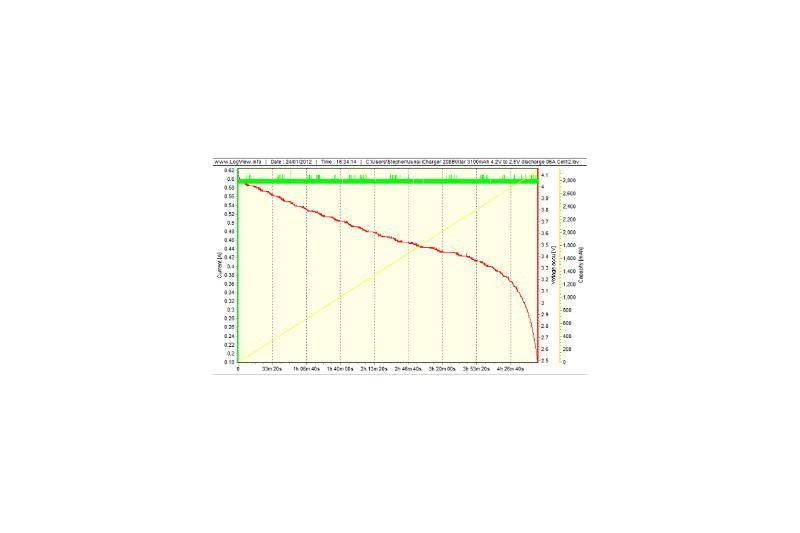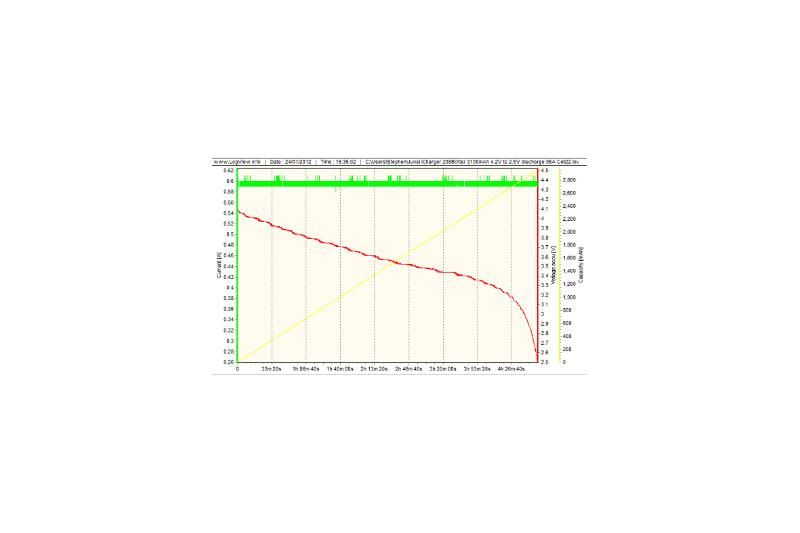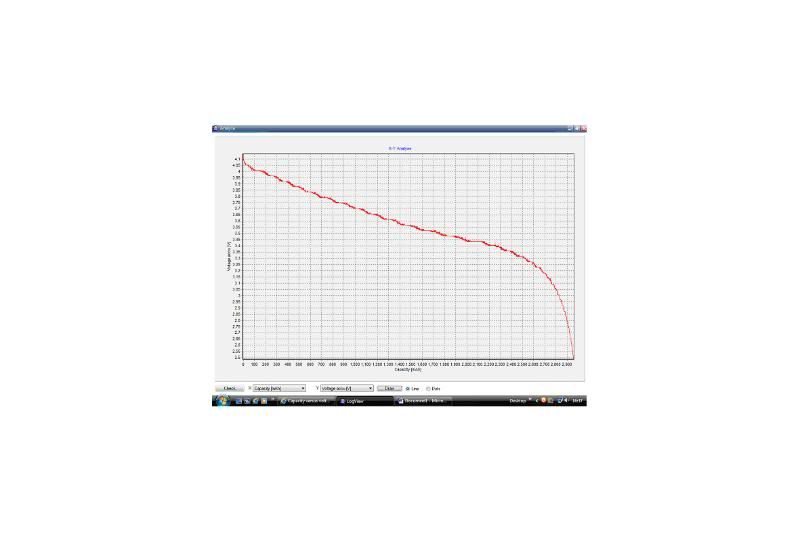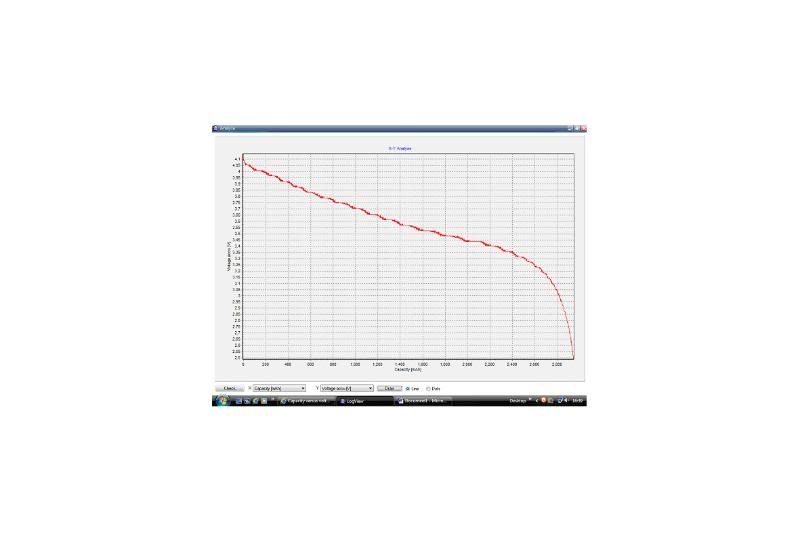Discussion in 'International Area' started by Stephen Wallace, Aug 21, 2011.

1. Apologies - my German isn't nearly good enough to read the directions for using LogView.I wondered if it were possible to plot a graph of capacity versus voltage when discharging a battery, having capacity on the x-axis rather than time.

I understand that I can plot capacity and voltage on the same graph, but the standard graph settings always show time on the x-axis, and capacity is shown as a diagonal line between voltage on the y-axis and time on the x-axis.

I also wondered if someone could quickly explain to me how to go about showing several discharge curves on one graph for comparison. For instance, if I had the discharge curves for four cells of the same type, from four separate discharge cycles, can I layer these plots on to the same graph?Many thanks :grin:
Stephen

Hi !

You can do it after the logging.
Just use Analysis -> Analyse x-y for this.

Click on check and select your axis. Click an Draw and your are done.

Just rightclick in the Graph settings on one curve. Select "Copy to compare Chart".
Repeat it with your other datasets.
Now select Compare Curve under the chart view.
3. Dominik,

I shall give both those things a try and see how I get on.

Thanks again
Stephen
4. Dominik,

Thanks again for your previous assistance - I have now been able to plot a discharge curve, and then change the graph axes to produce a graph of voltage against capacity.

I have also been able to carry out a number of discharges, and combine the plots in to one graph.

What I haven't managed to do is combine a number of plots on to one graph, and then change the X-axis to capacity. Should that be possible? After using the 'compare' function, it doesn't seem to be possible to use the 'Analysis' function to change the axes of the graph.

Should I be using 'Analysis' on each plot first, then using the 'Compare' function after? If so, would I use Graph -> Save As to save the plts after changing the axes and prior to using 'Compare', or would it be File -> Save As?

Many thanks
Stephen
5. Apologies for bumping my own thread, but I haven't been able to work this out for myself.

I am still trying to create a plot with a comparison of two discharge curves, AND the x-axis changed from time to capacity.

I have been able to take one discharge curve, and then changed the x-axis to capacity. I have also been able to compare two discharge curves (with time on the x-axis) and create one comparison plot. However, it seems like in either case, this is as far as I can go. I haven't been able to compare two plots with capacity on the x-axis, or to create a comparison plot and then change the x-axis to capacity.

So for instance, if I had the following two discharge plots: -I am able to use the analysis function on each plot individually, to get capacity on the x-axis: -I can use the compare curve function to get both plots on the same graph: -But is there anyway to then take that last comparison graph and then change the x-axis from time to capacity?

Many thanks
Stephen